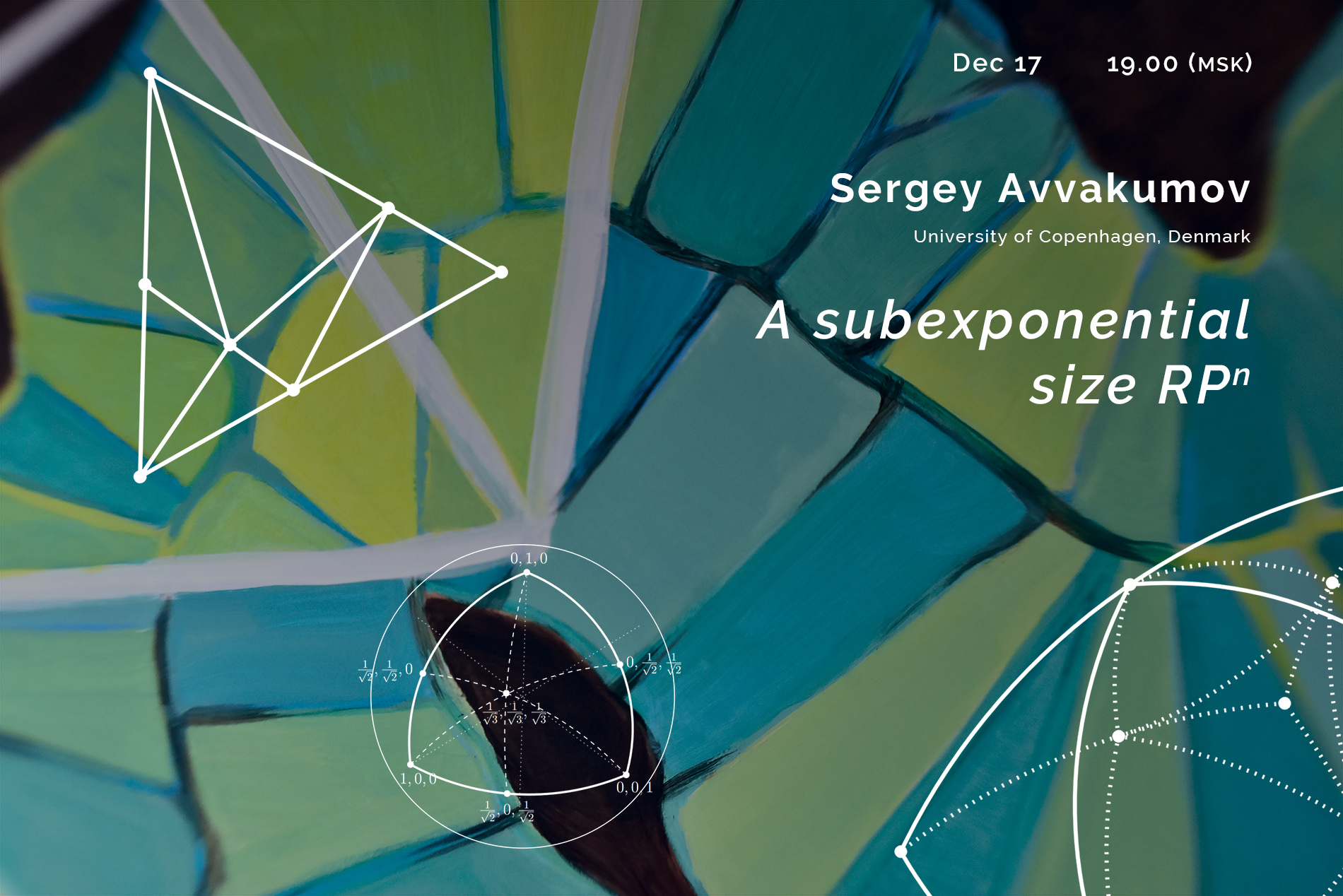# Sergey Avvakumov “A subexponential size RP^n” | Big SeminarDecember 17, 2020
19.00 MSK (UTC +3)
Talk on Big Seminar

# Sergey Avvakumov «A subexponential size ${\mathbb R}P^n$»

Sergey Avvakumov from University of Copenhagen gave the talk «A subexponential size ${\mathbb R}P^n$» on the labs' Big Seminar.

The talk will be held in zoom
Meeting ID: 279-059-822
Password: first 6 decimal places of $\pi$ after the decimal point

You can also write to Alexander Polyanskii (alexander.polyanskii@yandex.ru) or to Maksim Zhukovskii (zhukmax@gmail.com) if you want to be added to mailing list.

Abstract:

A practical way to encode a manifold is to triangulate it. Among all possible triangulations it makes sense to look for the minimal one, which for the purpose of this talk means using the least number of vertices.

Consider now a family of manifolds such as $S^n$, ${\mathbb R}P^n$, $SO_n$, etc. A natural question is how the size of the minimal triangulation depends on $n$? Surprisingly, except for the trivial case of $S^n$, our best lower and upper bounds are very far apart.

For ${\mathbb R}P^n$ the current best lower and upper bounds are around $n^2$ and $\phi^n$, respectively, where $\phi$ is the golden ratio. In this talk I will present the first triangulation of ${\mathbb R}P^n$ with a subexponential, $e^{(\frac{1}{2}+o(1))\sqrt{n}{\log n}}$, number of vertices. I will also state several open problems related to the topic.

This is a joint work with Karim Adiprasito and Roman Karasev.

## Watch the video:

Everyone is invited to attend. The language of the lecture is English. The event is aimed at master and graduate students, as well as researchers in the field of combinatorics.